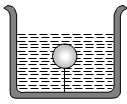A silver ingot weighing 2.1 kg is held by a string so as to be completely immersed in a liquid of relative density 0.8. The relative density of silver is 10.5. The tension in the string in kg-wt is
(a) 1.6                                             (b) 1.94
(c) 3.1                                             (d) 5.25

Concept Questions :-

Properties of fluids
High Yielding Test Series + Question Bank - NEET 2020

Difficulty Level:

Two solids A and B float in water. It is observed that A floats with half its volume immersed and B floats with 2/3 of its volume immersed. Compare the densities of A and B

(a) 4:3                         (b) 2:3

(c) 3:4                         (d) 1:3

Concept Questions :-

Archimedes principle
High Yielding Test Series + Question Bank - NEET 2020

Difficulty Level:

The fraction of a floating object of volume ${\mathrm{V}}_{0}$ and density ${\mathrm{d}}_{0}$ above the surface of a liquid of density d will be

(a) $\frac{{\mathrm{d}}_{0}}{\mathrm{d}}$                                       (b) $\frac{{\mathrm{dd}}_{0}}{\mathrm{d}+{\mathrm{d}}_{0}}$

(c) $\frac{\mathrm{d}-{\mathrm{d}}_{0}}{\mathrm{d}}$                                   (d) $\frac{{\mathrm{dd}}_{0}}{\mathrm{d}-{\mathrm{d}}_{0}}$

Concept Questions :-

Archimedes principle
High Yielding Test Series + Question Bank - NEET 2020

Difficulty Level:

Pressure applied to an enclosed fluid is transmitted undiminished to every portion of the fluid and the walls of the containing vessel. This law was first formulated by:

(a) Bernoulli                                (b) Archimedes
(c) Boyle                                     (d) Pascal

Concept Questions :-

Pressure

Difficulty Level:

A body is just floating on the surface of a liquid. The density of the body is same as that of the liquid. The body is slightly pushed down. What will happen to the body ?
(a) It will slowly come back to its earlier position

(b) It will remain submerged, where it is left

(c) It will sink

(d) It will come out violently

Concept Questions :-

Archimedes principle
High Yielding Test Series + Question Bank - NEET 2020

Difficulty Level:

A cork is submerged in water by a spring attached to the bottom of a bowl. When the bowl is kept in an elevator moving with acceleration downwards, the length of spring
(a) Increases                                          (b) Decreases
(c) Remains unchanged                           (d) None of these

Concept Questions :-

Archimedes principle
High Yielding Test Series + Question Bank - NEET 2020

Difficulty Level:

A solid sphere of density $\mathrm{\eta }$ ( > 1) times lighter than water is suspended in a water tank by a string tied to its base as shown in fig. If the mass of the sphere is m then the tension in the string is given by(a) $\left(\frac{\mathrm{\eta }-1}{\mathrm{\eta }}\right)\mathrm{mg}$                              (b) $\mathrm{\eta mg}$

(c) $\frac{mg}{\mathrm{\eta }}$                                        (d) $\left(\mathrm{\eta }-1\right)\mathrm{mg}$

Concept Questions :-

Archimedes principle
High Yielding Test Series + Question Bank - NEET 2020

Difficulty Level:

A hollow sphere of volume V is floating on water surface with half immersed in it. What should be the minimum volume of water poured inside the sphere so that the sphere now sinks into the water ?
(a) V/2                        (b) V/3

(c) V/4                        (d) V

Concept Questions :-

Archimedes principle
High Yielding Test Series + Question Bank - NEET 2020

Difficulty Level:

A ball whose density is  falls into water from a height of 9 cm . To what depth does the ball sink
(a) 9 cm                                    (b) 6 cm
(c) 4.5 cm                                 (d) 2.25 cm

Concept Questions :-

Archimedes principle
High Yielding Test Series + Question Bank - NEET 2020

Difficulty Level:

Two solids A and B float in water. It is observed that A floats with $\frac{1}{2}$ of its body immersed in water and B floats with $\frac{1}{4}$ of its volume above the water level. The ratio of the density of A to that of B is
1. 4 : 3                                  2. 2 : 3
3. 3 : 4                                  4. 1 : 2

Concept Questions :-

Archimedes principle